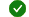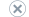# Utility nodes

Applies to:Visual StudioVisual Studio for MacVisual Studio Code

In the Shader Designer, utility nodes represent common, useful shader calculations that don't fit neatly into the other categories. Some utility nodes perform simple operations such as appending vectors together or choosing results conditionally, and others perform complex operations such as computing lighting contributions according to popular lighting models.

## Utility node reference

Node Details Properties
Append Vector Creates a vector by appending the specified inputs together.

Input:

`Vector`: `float`, `float2`, or `float3`
The values to append to.

`Value to Append`: `float`
The value to append.

Output:

`Output`: `float2`, `float3`, or `float4` depending on the type of input `Vector`
The new vector.
None
Fresnel Computes the Fresnel fall-off based on the specified surface normal.

The Fresnel fall-off value expresses how closely the surface normal of the current pixel coincides with the view vector. When the vectors are aligned, the result of the function is 0; the result increases as the vectors become less similar, and reaches its maximum when the vectors are orthogonal. You can use this to make an effect more or less apparent based on the relationship between the orientation of the current pixel and the camera.

Input:

`Surface Normal`: `float3`
The surface normal of the current pixel, defined in the current pixel's tangent space. You can use this to perturb the apparent surface normal, as in normal mapping.

Output:

`Output`: `float`
The reflectivity of the current pixel.
Exponent
The exponent that's used to calculate the Fresnel fall-off.
If Conditionally chooses one of three potential results per component. The condition is defined by the relationship between two other specified inputs.

For each component of the result, the corresponding component of one of the three potential results is chosen, based on the relationship between the corresponding components of the first two inputs.

Input:

`X`: `float`, `float2`, `float3`, or `float4`
The left-hand side value to compare.

`Y`: same type as input `X`
The right-hand side value to compare.

`X > Y`: same type as input `X`
The values that are chosen when `X` is greater than `Y`.

`X = Y`: same type as input `X`
The values that are chosen when `X` is equal to `Y`.

`X < Y`: same type as input `X`
The values that are chosen when `X` is less than `Y`.

Output:

`Output`: `float3`
The chosen result, per component.
None
Lambert Computes the color of the current pixel according to the Lambert lighting model, by using the specified surface normal.

This color is the sum of ambient color and diffuse lighting contributions under direct lighting. Ambient color approximates the total contribution of indirect lighting, but looks flat and dull without the help of additional lighting. Diffuse lighting helps add shape and depth to an object.

Input:

`Surface Normal`: `float3`
The surface normal of the current pixel, defined in the current pixel's tangent space. You can use this to perturb the apparent surface normal, as in normal mapping.

`Diffuse Color`: `float3`
The diffuse color of the current pixel, typically the Point Color. If no input is provided, the default value is white.

Output:

`Output`: `float3`
The diffuse color of the current pixel.
None

You can use this to remove specific color channels from a color value, or to prevent specific components from having an effect on subsequent calculations.

Input:

`Vector`: `float4`

Output:

`Output`: `float4`
Red / X
False to mask out the red (x) component; otherwise, True.

Green / Y
False to mask out the green (y) component; otherwise, True.

Blue / Z
False to mask out the blue (z) component; otherwise, True.

Alpha / W
False to mask out the alpha (w) component; otherwise, True.
Reflection Vector Computes the reflection vector for the current pixel in tangent space, based on the camera position.

You can use this to calculate reflections, cubemap coordinates, and specular lighting contributions

Input:

`Tangent Space Surface Normal`: `float3`
The surface normal of the current pixel, defined in the current pixel's tangent space. You can use this to perturb the apparent surface normal, as in normal mapping.

Output:

`Output`: `float3`
The reflection vector.
None
Specular Computes the specular lighting contribution according to the Phong lighting model, by using the specified surface normal.

Specular lighting gives a shiny, reflective appearance to an object, for example, water, plastic, or metals.

Input:

`Surface Normal`: `float3`
The surface normal of the current pixel, defined in the current pixel's tangent space. You can use this to perturb the apparent surface normal, as in normal mapping.

Output:

`Output`: `float3`
The color contribution of specular highlights.
None